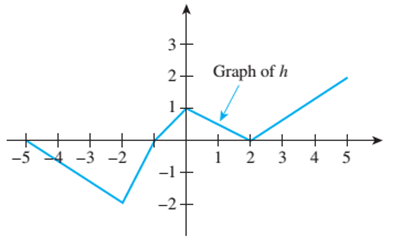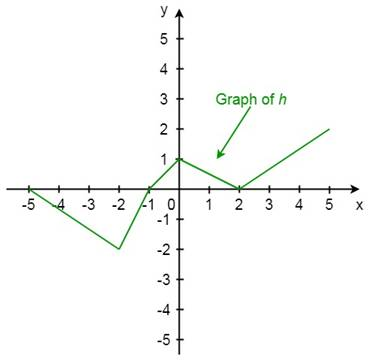Chapter 11.1, Problem 23ES### Discrete Mathematics With Applicat...

5th Edition
EPP + 1 other
ISBN: 9781337694193

#### Solutions

Chapter
Section### Discrete Mathematics With Applicat...

5th Edition
EPP + 1 other
ISBN: 9781337694193
Textbook Problem
1 views

# Let h be the function whose graph is shown below. Sketch the graph of 2h.To determine

Let h be the funciton whose graph is show below. Draw the graph of 2hExplanation

Given information:

2h

Concept used:

2f(x)=2(f(x))

Calculation:

Objective is to draw the graph of 2h.

Observe that the graph has 5 piecewise straight lines starting from left to right.

Part 1:(5,0),(2,2)

The line is as follows.

y0=202+5(x+5)y=23(x+5)

Part 2:(2,2) and (1,0)

y+2=0+21+2(x+2)y+2=2(x+2)y=2x+2

Part 3:(1,0) and (0,1)

y0=100+1(x+1)y=x+1

Part 4:(0,1) and (2,0).

y1=0120(x0)y=12x+1

Part 5:(2,0) and (5,2)

y0=2052(x2)y=23(x2)

Using increase the above 5 parts by 3 times

### Still sussing out bartleby?

Check out a sample textbook solution.

See a sample solution

#### The Solution to Your Study Problems

Bartleby provides explanations to thousands of textbook problems written by our experts, many with advanced degrees!

Get Started